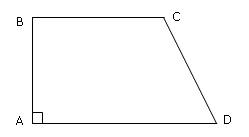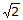### TANCET Past Question papers, TANCET 2008 Quant Section, TANCET old papers : Ascent Education TANCET Classes

 Home TANCET Classes TANCET Study Material XAT '14 Class CAT '13 Classes GMAT Classes
 TANCET 2018 - Courses TANCET MBA Classes Chennai TANCET Study Material TANCET FAQ TANCET MBA College List TANCET Toppers TANCET Testimonials TANCET Y! Groups TANCET Previous Qn Papers GMAT ClassesWeekend and Weekday classroom courses for the GMAT at Chennai. Faculty includes IIM and ISB graduates. Top score 770. Admits to Harvard, Stanford, ISB and other leading B schools.CAT Classroom ProgramWeekend classes for CAT @ Chennai by CAT serial 100 percentiler.Other Courses GRE Classes SAT Classes Other Links CAT XAT TANCET Questions Business Partnerships Careers @ Ascent Contact Us +91 44 4500 8484 +91 96000 48484 ascent@ascenteducation.com Postal Address Facebook / Twitter
You are here: Home » TANCET Previous Year Papers » TANCET 2008 Math Section

# TANCET Past Papers : TANCET 2008 Quant

1. Question 1 : Linear Equation : An owner of a pizza stand sold small slices of pizza for Rs. 150 each and large slices for Rs. 250 each. One night he sold 5000 slices, for a total of Rs. 10.50 lakh. How many small slices were sold?

2. Question 2 : Framing Equations : Jack has three more cards than Bill. Together they have 47 cards. If x represents the number of cards Bill has, then an equation that can be used to determine the number of cards each one has is

3. Question 3 : Number Properties - Fractions : If two fractions, each of which has a value between 0 and 1, are multiplied together, the product will be:

4. Question 4 : Work Time : A father can do a certain job in x hours. His son takes twice as long to do the job. Working together, they can do the job in 6 hours. How many hours does the father take to do the job?

5. Question 5 : Mensuration : If x units are added to the length of the radius of a circle, what is the number of units by which the circumference of the circle is increased?

6. Question 6 : Percents, Comparison : John weighs twice as much as Maria. Marcia's weight is 60% of Bob's weight. Dave weighs 50% of Lee's weight. Lee weighs 190% of John's weight. Which of these 5 persons weighs the least?

7. Question 7 : Mathematical Expressions : There were P people in a room when a meeting started. Q people left the room during the first hour, while R people entered the room during the same time. What expression gives the number of people in the room after the first hour as a percentage of the number of people in the room who have been there since the meeting started?

8. Question 8 : Mathematical Expressions : It costs Rs. x each to make the first thousand copies of a compact disk and Rs. y to make each subsequent copy. If z is greater than 1,000, how many Rupees will it cost to make z copies of the compact disk?

9. Question 9 : Geometry - Right Triangles : If the shaded area is one half the area of triangle ABC and angle ABC is right angle, then the length of line segment AD is10. Question 10 : Speed, Distance & Time : A plane flying north at 500 kmph passes over a city at 12 noon. A plane flying east at the same attitude passes over the same city at 12.30 pm. The plane is flying east at 400 kmph. To the nearest hundred km, how far apart are the two planes at 2 pm?

11. Question 11 : Framing Expressions : Profits : A clothing manufacturer has determined that she can sell 100 suits a week at a selling price of Rs. 200 each. For each rise of Rs. 4 in the selling price she will sell 2 less suits a week. If she sells the suits for Rs. x each, how many rupees a week will she receive from the sales of the suits?

12. Question 12 : Framing Expressions : Set Theory : The schedule of G first year students was inspected. It was found that M were taking a Mathematics course, L were taking a Language course and B were taking both a Mathematics course and a Language course. Which of the following expression gives the percentage of the students whose schedule was inspected who were taking neither a mathematics course nor a language course?

13. Question 13 : Speed, Distance & Time : A plane flying north at 500 kmph passes over a city at 12 noon. A plane flying east at the same attitude passes over the same city at 12.30 pm. The plane is flying east at 400 kmph. To the nearest hundred km, how far apart are the two planes at 2 pm?

14. Question 14 : Percents »  Joan started work 2 years ago. Her starting salary was half of Mike's salary at that time. Each year since then Joan and Mike have received a rise of 10% in their respective salary. What percentage (to the nearest percent) of Mike's current salary is Joan's current salary?

15. Question 15 : Mensuration - Solid Geometry »  ABCD has area equal to 28. BC is parallel to AD. BA is perpendicular to AD. If BC is 6 and AD is 8, then what is CD?16. Question 16 : Mensuration - Solid Geometry »  Which of the following figures has the largest area?
1. A circle of radius2. An equilateral triangle whose sides each have length 4
3. A triangle whose sides have lengths 3, 4 and 5

17. Question 17 : Mathematical Expressions - equations »  A charity solicited P persons over the phone who agreed to an average pledge of Rs.R each. Q of these people who had pledged an average of Rs. S each never sent in the pledged amount. Which of the following expressions represents the percentage of pledged money that the charity received?
18. Question 18 : Number Theory »  If x, y, z are chosen from the three numbers, -3,and 2, what is the largest possible value of the expressionz2?Answer and Explanation »Add to del.icio.usStumble Itdigg this